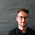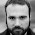## Tuesday, April 12, 2016

### Approaches to quantum gravity

Again as a part of a lecture series in preparation, I compiled a list of the currently largest existing research programmes aimed at finding a quantum theory of gravity (while unfortunately omitting some smaller, yet very interesting, approaches). A much more extensive account is given in here. For an historical overview, I recommend this paper.

Semiclassical gravity

Semiclassical gravity is a first step towards quantum gravity, where matter fields are treated using full quantum field theory, while the geometry remains classical. However, semiclassical gravity goes beyond quantum field theory on curved spacetimes: the energy-momentum tensor determining the spacetime geometry via Einstein’s equations is taken to be the expectation value of the QFT energy-momentum tensor. The state in which this expectation value is evaluated in turn depends on the geometry, and one has to find a self-consistent solution. Many of the original problems of semi-classical gravity have been addressed recently and the theory can be applied in practise.

Ordinary quantum field theory

The most straight forward approach to quantising gravity itself is to use ordinary perturbative quantum field theory to quantise the deviation of the metric from a given background. While it turned out that general relativity is non-renormalisable in the standard picture, it is possible to use effective field theory techniques in order to have a well-defined notion of perturbative quantum gravity up to some energy scale lower than the Planck scale. Ordinary effective field theory thus can describe a theory of quantum gravity at low energies, whereas it does not aim to understand quantum gravity in extreme situations, such as cosmological or black hole singularities.

Supergravity

Supergravity has been invented with the hope of providing a unified theory of matter and geometry which is better behaved in the UV than Einstein gravity. As opposed to standard supersymmetric quantum field theories, supergravity exhibits a local supersymmetry relating matter and gravitational degrees of freedom. In the symmetry algebra, this fact is reflected by the generator of local supersymmetries squaring to spacetime-dependent translations, i.e. general coordinate transformations.

While the local supersymmetry generally improved the UV behaviour of the theories, it turned out that also supergravity theories were non-renormalisable. The only possible exception seems to be N = 8 supergravity in four dimensions, which is known to be finite at four loops, but it is unclear what happens beyond. Nowadays, supergravity is mostly considered within string theory, where 10-dimensional supergravity appears as a low energy limit. Due to its finiteness properties, string theory can thus be considered as a UV-completion for supergravity. Moreover, 11-dimensional supergravity is considered as the low-energy limit of M-theory, which is conjectured to have the 5 different string theories as specific limits.

Asymptotic safety

The underlying idea of asymptotic safety is that while general relativity is perturbatively non- renormalizable, its renormalisation group flow might possess a non-trivial fixed point where the couplings are finite. In order to investigate this possibility, the renormalisation group equations need to be solved. For this, the “theory space”, i.e. the space of all action functionals respecting the symmetries of the theory, has to be suitably truncated in practise. Up to now, much evidence has been gathered that general relativity is asymptotically safe, including matter couplings, however always in certain truncations, so that the general viability of the asymptotic safety scenario has not been rigorously established so far. Also, one mostly works in the Euclidean. At microscopic scales, one finds a fractal-like effective spacetime and a reduction of the (spectral) dimension from 4 to 2 (or 3/2, which is favoured by holographic arguments, depending on the calculation). Moreover, a derivation of the Higgs mass has been given in the asymptotic safety scenario, correctly predicting it before its actual measurement.

Canonical quantisation: Wheeler-de Witt

The oldest approach to full non-perturbative quantum gravity is the Wheeler-de Witt theory, i.e. the canonical quantisation of the Arnowitt-Deser-Misner formulation of general relativity. In this approach, also known as quantum geometrodynamics, one uses the spatial metric and its conjugate momentum as canonical variables. The main problems of the Wheeler-de Witt approach are of mathematical nature: the Hamiltonian constraint operator is extremely difficult to define due to its non-linearity and a Hilbert space to support is is not known. It is therefore strongly desirable to find new canonical variables for general relativity in which the quantisation is more tractable. While the so called “problem of time”, i.e. the absence of a physical background notion of time in general relativity, is present both in the quantum and the classical theory, possible ways to deal with it are known and continuously developed.

Euclidean quantum gravity

In Euclidean quantum gravity, a Wick rotation to Euclidean space is performed, in which the gravity path integral is formulated as a path integral over all metrics. Most notably, this approach allows to extract thermodynamic properties of black holes. In practise, the path integral is often approximated by the exponential of the classical on-shell action. Its main problematic aspect is that the Wick rotation to Euclidean space is well defined only for a certain limited class of spacetimes, and in particular dynamical phenomena are hard to track. An overview can be found here.

Causal dynamical triangulations

Causal dynamical triangulations (CDT) is a non-perturbative approach to rigorously define a path integral for general relativity based on a triangulation. It grew out of the Euclidean dynamical triangulations programme, which encountered several difficulties in the 90’s, by adding a causality constraint on the triangulations. The path integral is then evaluated using Monte Carlo techniques. The phase diagram of CDT in four dimensions exhibits three phases, one of which is interpreted as a continuum four-dimensional universe. Moreover, the transition between this phase and one other phase is of second order, hinting that one might be able to extract a genuine continuum limit. More recently, also Euclidean dynamical triangulations has been reconsidered and evidence for a good semiclassical limit has been reported.

String theory

String theory was initially conceived as a theory of the strong interactions, where the particle concept is replaced by one-dimensional strings propagating in some background spacetime. It was soon realised that the particle spectrum of string theory includes a massless spin 2 excitation, which is identified as the graviton. Moreover, internal consistency requirements demand (in lowest order) the Einstein equations to be satisfied for the background spacetimes. String theory thus is automatically also a candidate for a quantum theory of gravity. The main difference of string theory with the other approaches listed here is thus that the quantisation of gravity is achieved via unification of gravity with the other forces of nature, as opposed to considering the problem of quantum gravity separately.

The main problem of string theory is that it seems to predict the wrong spacetime dimension: 26 for bosonic strings, 10 for supersymmetric strings, and 11 in the case of M-theory. In order to be compatible with the observed 3 + 1 dimensions at the currently accessible energies, one therefore needs to compactify some of the extra dimensions. In this process, a large amount of arbitrariness is introduced and it has remained an open problem to extract predictions from string theory which are independent of the details of the compactification. Also, our knowledge about full non-perturbative string theory is limited, with the main exceptions of D-branes and using AdS/CFT as a definition of string theory.

Gauge / gravity

The gauge / gravity correspondence, also known as AdS/CFT, has grown out of string theory, but was later recognised to be applicable more widely. Its statement is a (in most cases conjectured) duality between a quantum gravity theory on some class of spacetimes, and a gauge theory living on the boundary of the respective spacetime. Once a complete dictionary between gravity and field theory computations is known, one can in principle use the gauge / gravity correspondence as a definition of quantum gravity on that class of spacetimes.

The main problem of gauge / gravity as a tool to understand quantum gravity is the lack of a complete dictionary between the two theories, in particular for local bulk observables. Also, it is usually very hard to find gauge theory duals of realistic gravity theories already at the classical gravity level (i.e. in an appropriate field theory limit), and many known examples are very special supersymmetric theories.

Loop quantum gravity

Loop quantum gravity originally started as a canonical quantisation of general relativity, in the spirit of the Wheeler-de Witt approach, however based on connection variables parametrising the phase space of general relativity, e.g. the Ashtekar-Barbero variables based on the gauge group SU(2). The main advantage of these variables is that one can rigorously define a Hilbert space (containing Wilson-"loops" of the connection, hence the name) and quantise the Hamiltonian constraint. The application of the main technical and conceptual ideas of loop quantum gravity to quantum cosmology resulted in the subfield of loop quantum cosmology, which offers a quantum gravity resolution of the big bang singularity and successfully makes contact to observation.

The main problem of loop quantum gravity is to obtain general relativity in a suitably defined classical limit. In other words, the fundamental quantum geometry present in loop quantum gravity has to be coarse grained in order to yield a smooth classical spacetime, while the behaviour of matter fields coupled to the theory should be dictated by standard quantum field theory on curved spacetimes in this limit. The situation is thus roughly the opposite of that in string theory. Also, it has not been possible so far to fully constrain the regularisation ambiguities that one encounters in quantising the Hamiltonian constraint. In order to cope with these issues, a path integral approach, known as spin foams, has been developed, as well as the group field theory approach, which is well suited for dealing with the question of renormalisation.

1.Nice review! In the last section an "of" is missing in the sentence "The application the main technical and conceptual ideas". In addition, I think a short explanation is missing on why loop quantum gravity is called LOOP quantum gravity at all.

Best wishes,

Jakob
http://JakobSchwichtenberg.com/

1.Dear Jakob,

thanks a lot for your comment. I fixed the typo and added a brief explanation for the origins of the name. It originated in the time before it was realised that one needs to go beyond Wilson-loops to have non-vanishing volumes, i.e. to Wilson-"graphs", but it got stuck.

2.Hi,

CDT was misspelled as "casual" DT instead of "causal".

Also in asymptotic safety you (almost) always go Euclidean.

Finally, in asympt. safety spectral dimension apparently goes to 3/2, and not 2 as previously thought. This was confirmed in CDT and very recently Euclidean Dynamical Triangulations (EDT). Note that this result is relevant as it bypasses one usual apriori counterargument against QFTs of gravity.

3.Hi Vedran,

thanks a lot for your input. I incorporated it in the post.

4.Nice review! I would like to point out that the described approaches are all based on the assumption that the short-distance topological structure of the spacetime is given by a smooth manifold. This is a logical possibility, but somehow I find it difficult to believe. Note that the approaches you refer to as "smaller" are precisely those where the smooth manifold structure is abandoned and one uses a non-commutative manifold, or a discrete set, or a piecewise linear manifold, etc ... Even in the case of CDT, although the theory is defined in the picewise linear manifold case (a triangulation), the goal is to obtain the smooth-manifold limit. More than 20 years ago Chris Isham has described this problem in one of his QG reviews: one starts with a smooth manifold M and a metric g, so the simplest thing is to replace (M,g) with (M,g*), where g* is an operator. A more general quantization is to replace (M,g) with (M*,g*) where M* and g* are some quantum generalizations of M and g.